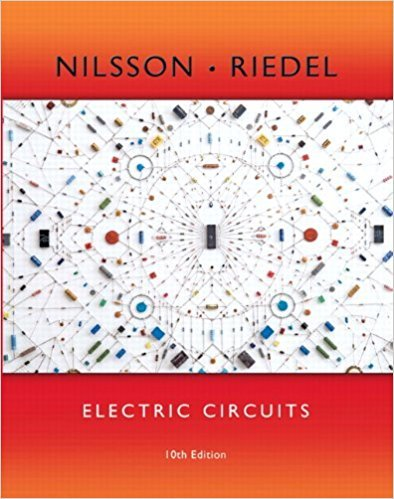×
×

# Solved: The op amp in the circuit in Fig. P5.4 is ideal. a) Calculate if and b)ISBN: 9780133760033 390

## Solution for problem 5.4 Chapter 5

Electric Circuits | 10th Edition

• Textbook Solutions
• 2901 Step-by-step solutions solved by professors and subject experts
• Get 24/7 help from StudySoup virtual teaching assistantsElectric Circuits | 10th Edition

4 5 1 244 Reviews
31
3
Problem 5.4

The op amp in the circuit in Fig. P5.4 is ideal. a) Calculate if and b) Calculate if and c) Calculate if and d) Calculate if and v vb = 1 V. e) Calculate if andf) If , specify the range of such thatthe amplifier does not saturate.

Step-by-Step Solution:
Step 1 of 3

ENGR 121 B Lecture Notes for 9/19/2016 Spencer Kociba Summary of Lecture: beginning to understand the vocabulary and functions in MATLAB and how we will use MATLAB and BlackBoard Learn to turn in assignments, take quizzes, etc. Goal of the course: learn to THINK like a programmer aka step by step problem solving. Problem solving techniques:...

Step 2 of 3

Step 3 of 3

##### ISBN: 9780133760033

This textbook survival guide was created for the textbook: Electric Circuits, edition: 10. Since the solution to 5.4 from 5 chapter was answered, more than 238 students have viewed the full step-by-step answer. Electric Circuits was written by and is associated to the ISBN: 9780133760033. The answer to “The op amp in the circuit in Fig. P5.4 is ideal. a) Calculate if and b) Calculate if and c) Calculate if and d) Calculate if and v vb = 1 V. e) Calculate if andf) If , specify the range of such thatthe amplifier does not saturate.” is broken down into a number of easy to follow steps, and 48 words. This full solution covers the following key subjects: . This expansive textbook survival guide covers 107 chapters, and 1357 solutions. The full step-by-step solution to problem: 5.4 from chapter: 5 was answered by , our top Engineering and Tech solution expert on 03/13/18, 07:48PM.

Unlock Textbook Solution